Intermediate Algebra

# 1.5Properties of Real Numbers

Intermediate Algebra1.5 Properties of Real Numbers

### Learning Objectives

By the end of this section, you will be able to:
• Use the commutative and associative properties
• Use the properties of identity, inverse, and zero
• Simplify expressions using the Distributive Property

### Be Prepared 1.5

A more thorough introduction to the topics covered in this section can be found in the Elementary Algebra chapter, Foundations.

### Use the Commutative and Associative Properties

The order we add two numbers doesn’t affect the result. If we add $8+98+9$ or $9+8,9+8,$ the results are the same—they both equal 17. So, $8+9=9+8.8+9=9+8.$ The order in which we add does not matter!

Similarly, when multiplying two numbers, the order does not affect the result. If we multiply $9·89·8$ or $8·98·9$ the results are the same—they both equal 72. So, $9·8=8·9.9·8=8·9.$ The order in which we multiply does not matter!

These examples illustrate the Commutative Property.

### Commutative Property

$of AdditionIfaandbare real numbers, thena+b=b+a. of MultiplicationIfaandbare real numbers, thena·b=b·a. of AdditionIfaandbare real numbers, thena+b=b+a. of MultiplicationIfaandbare real numbers, thena·b=b·a.$

When adding or multiplying, changing the order gives the same result.

The Commutative Property has to do with order. We subtract $9−89−8$ and $8−98−9$, and see that $9−8≠8−9.9−8≠8−9.$ Since changing the order of the subtraction does not give the same result, we know that subtraction is not commutative.

Division is not commutative either. Since $12÷3≠3÷12,12÷3≠3÷12,$ changing the order of the division did not give the same result. The commutative properties apply only to addition and multiplication!

Subtraction and division are not commutative.

When adding three numbers, changing the grouping of the numbers gives the same result. For example, $(7+8)+2=7+(8+2),(7+8)+2=7+(8+2),$ since each side of the equation equals 17.

This is true for multiplication, too. For example, $(5·13)·3=5·(13·3),(5·13)·3=5·(13·3),$ since each side of the equation equals 5.

These examples illustrate the Associative Property.

### Associative Property

$of AdditionIfa,b,andcare real numbers, then(a+b)+c=a+(b+c). of MultiplicationIfa,b,andcare real numbers, then(a·b)·c=a·(b·c). of AdditionIfa,b,andcare real numbers, then(a+b)+c=a+(b+c). of MultiplicationIfa,b,andcare real numbers, then(a·b)·c=a·(b·c).$

When adding or multiplying, changing the grouping gives the same result.

The Associative Property has to do with grouping. If we change how the numbers are grouped, the result will be the same. Notice it is the same three numbers in the same order—the only difference is the grouping.

We saw that subtraction and division were not commutative. They are not associative either.

$(10−3)−2≠10−(3−2)(24÷4)÷2≠24÷(4÷2) 7−2≠10−16÷2≠24÷2 5≠93≠12 (10−3)−2≠10−(3−2)(24÷4)÷2≠24÷(4÷2) 7−2≠10−16÷2≠24÷2 5≠93≠12$

When simplifying an expression, it is always a good idea to plan what the steps will be. In order to combine like terms in the next example, we will use the Commutative Property of addition to write the like terms together.

### Example 1.45

Simplify: $18p+6q+15p+5q.18p+6q+15p+5q.$

### Try It 1.89

Simplify: $23r+14s+9r+15s.23r+14s+9r+15s.$

### Try It 1.90

Simplify:$37m+21n+4m−15n.37m+21n+4m−15n.$

When we have to simplify algebraic expressions, we can often make the work easier by applying the Commutative Property or Associative Property first.

### Example 1.46

Simplify: $(513+34)+14.(513+34)+14.$

### Try It 1.91

Simplify: $(715+58)+38.(715+58)+38.$

### Try It 1.92

Simplify: $(29+712)+512.(29+712)+512.$

### Use the Properties of Identity, Inverse, and Zero

What happens when we add 0 to any number? Adding 0 doesn’t change the value. For this reason, we call 0 the additive identity. The Identity Property of Addition that states that for any real number $a,a+0=aa,a+0=a$ and $0+a=a.0+a=a.$

What happens when we multiply any number by one? Multiplying by 1 doesn’t change the value. So we call 1 the multiplicative identity. The Identity Property of Multiplication that states that for any real number $a,a·1=aa,a·1=a$ and $1·a=a.1·a=a.$

We summarize the Identity Properties here.

### Identity Property

$of AdditionFor any real numbera:a+0=a0+a=a 0is theadditive identity of MultiplicationFor any real numbera:a·1=a1·a=a 1is themultiplicative identity of AdditionFor any real numbera:a+0=a0+a=a 0is theadditive identity of MultiplicationFor any real numbera:a·1=a1·a=a 1is themultiplicative identity$

What number added to 5 gives the additive identity, 0? We know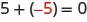The missing number was the opposite of the number!

We call $−a−a$ the additive inverse of $a.a.$ The opposite of a number is its additive inverse. A number and its opposite add to zero, which is the additive identity. This leads to the Inverse Property of Addition that states for any real number $a,a+(−a)=0.a,a+(−a)=0.$

What number multiplied by $2323$ gives the multiplicative identity, 1? In other words, $2323$ times what results in 1? We know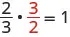The missing number was the reciprocal of the number!

We call $1a1a$ the multiplicative inverse of a. The reciprocal of a number is its multiplicative inverse. This leads to the Inverse Property of Multiplication that states that for any real number $a,a≠0,a·1a=1.a,a≠0,a·1a=1.$

We’ll formally state the inverse properties here.

### Inverse Property

$of AdditionFor any real numbera,a+(−a)=0 −ais theadditive inverseofa A number and itsoppositeadd to zero. of MultiplicationFor any real numbera,a≠0,a·1a=1. 1ais themultiplicative inverseofa. A number and itsreciprocalmultiply to one. of AdditionFor any real numbera,a+(−a)=0 −ais theadditive inverseofa A number and itsoppositeadd to zero. of MultiplicationFor any real numbera,a≠0,a·1a=1. 1ais themultiplicative inverseofa. A number and itsreciprocalmultiply to one.$

The Identity Property of addition says that when we add 0 to any number, the result is that same number. What happens when we multiply a number by 0? Multiplying by 0 makes the product equal zero.

What about division involving zero? What is $0÷3?0÷3?$ Think about a real example: If there are no cookies in the cookie jar and 3 people are to share them, how many cookies does each person get? There are no cookies to share, so each person gets 0 cookies. So, $0÷3=0.0÷3=0.$

We can check division with the related multiplication fact. So we know $0÷3=00÷3=0$ because $0·3=0.0·3=0.$

Now think about dividing by zero. What is the result of dividing 4 by $0?0?$ Think about the related multiplication fact: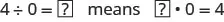Is there a number that multiplied by 0 gives $4?4?$ Since any real number multiplied by 0 gives 0, there is no real number that can be multiplied by 0 to obtain 4. We conclude that there is no answer to $4÷04÷0$ and so we say that division by 0 is undefined.

We summarize the properties of zero here.

### Properties of Zero

Multiplication by Zero: For any real number a,

$a·0=00·a=0The product of any number and 0 is 0.a·0=00·a=0The product of any number and 0 is 0.$

Division by Zero: For any real number a, $a≠0a≠0$

$0a=0Zero divided by any real number, except itself, is zero. a0is undefinedDivision by zero is undefined. 0a=0Zero divided by any real number, except itself, is zero. a0is undefinedDivision by zero is undefined.$

We will now practice using the properties of identities, inverses, and zero to simplify expressions.

### Example 1.47

Simplify: $−84n+(−73n)+84n.−84n+(−73n)+84n.$

### Try It 1.93

Simplify: $−27a+(−48a)+27a.−27a+(−48a)+27a.$

### Try It 1.94

Simplify: $39x+(−92x)+(−39x).39x+(−92x)+(−39x).$

Now we will see how recognizing reciprocals is helpful. Before multiplying left to right, look for reciprocals—their product is 1.

### Example 1.48

Simplify: $715·823·157.715·823·157.$

### Try It 1.95

Simplify: $916·549·169.916·549·169.$

### Try It 1.96

Simplify: $617·1125·176.617·1125·176.$

The next example makes us aware of the distinction between dividing 0 by some number or some number being divided by 0.

### Example 1.49

Simplify: $0n+5,0n+5,$ where $n≠−5n≠−5$ $10−3p0,10−3p0,$ where $10−3p≠0.10−3p≠0.$

### Try It 1.97

Simplify: $0m+7,0m+7,$ where $m≠−7m≠−7$ $18−6c0,18−6c0,$ where $18−6c≠0.18−6c≠0.$

### Try It 1.98

Simplify: $0d−4,0d−4,$ where $d≠4d≠4$ $15−4q0,15−4q0,$ where $15−4q≠0.15−4q≠0.$

### Simplify Expressions Using the Distributive Property

Suppose that three friends are going to the movies. They each need $9.25—that’s 9 dollars and 1 quarter—to pay for their tickets. How much money do they need all together? You can think about the dollars separately from the quarters. They need 3 times$9 so $27 and 3 times 1 quarter, so 75 cents. In total, they need$27.75. If you think about doing the math in this way, you are using the Distributive Property.

### Distributive Property

$Ifa,b,andcare real numbers, thena(b+c)=ab+ac (b+c)a=ba+ca a(b−c)=ab−ac (b−c)a=ba−ca Ifa,b,andcare real numbers, thena(b+c)=ab+ac (b+c)a=ba+ca a(b−c)=ab−ac (b−c)a=ba−ca$

In algebra, we use the Distributive Property to remove parentheses as we simplify expressions.

### Example 1.50

Simplify: $3(x+4).3(x+4).$

### Try It 1.99

Simplify: $4(x+2).4(x+2).$

### Try It 1.100

Simplify: $6(x+7).6(x+7).$

Some students find it helpful to draw in arrows to remind them how to use the Distributive Property. Then the first step in Example 1.50 would look like this: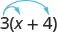### Example 1.51

Simplify: $8(38x+14).8(38x+14).$

### Try It 1.101

Simplify: $6(56y+12).6(56y+12).$

### Try It 1.102

Simplify: $12(13n+34).12(13n+34).$

Using the Distributive Property as shown in the next example will be very useful when we solve money applications in later chapters.

### Example 1.52

Simplify: $100(0.3+0.25q).100(0.3+0.25q).$

### Try It 1.103

Simplify: $100(0.7+0.15p).100(0.7+0.15p).$

### Try It 1.104

Simplify: $100(0.04+0.35d).100(0.04+0.35d).$

When we distribute a negative number, we need to be extra careful to get the signs correct!

### Example 1.53

Simplify: $−11(4−3a).−11(4−3a).$

### Try It 1.105

Simplify: $−5(2−3a).−5(2−3a).$

### Try It 1.106

Simplify: $−7(8−15y).−7(8−15y).$

In the next example, we will show how to use the Distributive Property to find the opposite of an expression.

### Example 1.54

Simplify: $−(y+5).−(y+5).$

### Try It 1.107

Simplify: $−(z−11).−(z−11).$

### Try It 1.108

Simplify: $−(x−4).−(x−4).$

There will be times when we’ll need to use the Distributive Property as part of the order of operations. Start by looking at the parentheses. If the expression inside the parentheses cannot be simplified, the next step would be multiply using the Distributive Property, which removes the parentheses. The next two examples will illustrate this.

### Example 1.55

Simplify: $8−2(x+3)8−2(x+3)$

### Try It 1.109

Simplify: $9−3(x+2).9−3(x+2).$

### Try It 1.110

Simplify: $7x−5(x+4).7x−5(x+4).$

### Example 1.56

Simplify: $4(x−8)−(x+3).4(x−8)−(x+3).$

### Try It 1.111

Simplify: $6(x−9)−(x+12).6(x−9)−(x+12).$

### Try It 1.112

Simplify: $8(x−1)−(x+5).8(x−1)−(x+5).$

All the properties of real numbers we have used in this chapter are summarized here.

 Commutative PropertyWhen adding or multiplying, changing the order gives the same result $of additionIfa,bare real numbers, thena+b=b+a of multiplicationIfa,bare real numbers, thena·b=b·aof additionIfa,bare real numbers, thena+b=b+a of multiplicationIfa,bare real numbers, thena·b=b·a$ Associative PropertyWhen adding or multiplying, changing the grouping gives the same result. $of additionIfa,b,andcare real numbers, then(a+b)+c=a+(b+c) of multiplicationIfa,b,andcare real numbers, then(a·b)·c=a·(b·c) of additionIfa,b,andcare real numbers, then(a+b)+c=a+(b+c) of multiplicationIfa,b,andcare real numbers, then(a·b)·c=a·(b·c)$ Distributive Property $Ifa,b,andcare real numbers, thena(b+c)=ab+ac (b+c)a=ba+ca a(b−c)=ab−ac (b−c)a=ba−ca Ifa,b,andcare real numbers, thena(b+c)=ab+ac (b+c)a=ba+ca a(b−c)=ab−ac (b−c)a=ba−ca$ Identity Property $of additionFor any real numbera:a+0=a 0is theadditive identity0+a=a of multiplicationFor any real numbera:a·1=a 1is themultiplicative identity1·a=a of additionFor any real numbera:a+0=a 0is theadditive identity0+a=a of multiplicationFor any real numbera:a·1=a 1is themultiplicative identity1·a=a$
 Inverse Property $of additionFor any real numbera,a+(−a)=0 −ais theadditive inverseofa A number and itsoppositeadd to zero. of multiplicationFor any real numbera,a≠0a·1a=1 1ais themultiplicative inverseofa A number and itsreciprocalmultiply to one. of additionFor any real numbera,a+(−a)=0 −ais theadditive inverseofa A number and itsoppositeadd to zero. of multiplicationFor any real numbera,a≠0a·1a=1 1ais themultiplicative inverseofa A number and itsreciprocalmultiply to one.$ Properties of Zero $For any real numbera,a·0=0 0·a=0 For any real numbera,a≠0,0a=0 For any real numbera,a0is undefined For any real numbera,a·0=0 0·a=0 For any real numbera,a≠0,0a=0 For any real numbera,a0is undefined$

### Section 1.5 Exercises

#### Practice Makes Perfect

Use the Commutative and Associative Properties

In the following exercises, simplify.

313.

$43 m + ( −12 n ) + ( −16 m ) + ( −9 n ) 43 m + ( −12 n ) + ( −16 m ) + ( −9 n )$

314.

$−22 p + 17 q + ( −35 p ) + ( −27 q ) −22 p + 17 q + ( −35 p ) + ( −27 q )$

315.

$3 8 g + 1 12 h + 7 8 g + 5 12 h 3 8 g + 1 12 h + 7 8 g + 5 12 h$

316.

$5 6 a + 3 10 b + 1 6 a + 9 10 b 5 6 a + 3 10 b + 1 6 a + 9 10 b$

317.

$6.8 p + 9.14 q + ( −4.37 p ) + ( −0.88 q ) 6.8 p + 9.14 q + ( −4.37 p ) + ( −0.88 q )$

318.

$9.6 m + 7.22 n + ( −2.19 m ) + ( −0.65 n ) 9.6 m + 7.22 n + ( −2.19 m ) + ( −0.65 n )$

319.

$−24 · 7 · 3 8 −24 · 7 · 3 8$

320.

$−36 · 11 · 4 9 −36 · 11 · 4 9$

321.

$( 5 6 + 8 15 ) + 7 15 ( 5 6 + 8 15 ) + 7 15$

322.

$( 11 12 + 4 9 ) + 5 9 ( 11 12 + 4 9 ) + 5 9$

323.

$17 ( 0.25 ) ( 4 ) 17 ( 0.25 ) ( 4 )$

324.

$36 ( 0.2 ) ( 5 ) 36 ( 0.2 ) ( 5 )$

325.

$[ 2.48 ( 12 ) ] ( 0.5 ) [ 2.48 ( 12 ) ] ( 0.5 )$

326.

$[ 9.731 ( 4 ) ] ( 0.75 ) [ 9.731 ( 4 ) ] ( 0.75 )$

327.

$12 ( 5 6 p ) 12 ( 5 6 p )$

328.

$20 ( 3 5 q ) 20 ( 3 5 q )$

Use the Properties of Identity, Inverse and Zero

In the following exercises, simplify.

329.

$19 a + 44 − 19 a 19 a + 44 − 19 a$

330.

$27 c + 16 − 27 c 27 c + 16 − 27 c$

331.

$1 2 + 7 8 + ( − 1 2 ) 1 2 + 7 8 + ( − 1 2 )$

332.

$2 5 + 5 12 + ( − 2 5 ) 2 5 + 5 12 + ( − 2 5 )$

333.

$10 ( 0.1 d ) 10 ( 0.1 d )$

334.

$100 ( 0.01 p ) 100 ( 0.01 p )$

335.

$3 20 · 49 11 · 20 3 3 20 · 49 11 · 20 3$

336.

$13 18 · 25 7 · 18 13 13 18 · 25 7 · 18 13$

337.

$0u−4.99,0u−4.99,$ where $u≠4.99u≠4.99$

338.

$0÷(y−16),0÷(y−16),$ where $x≠16x≠16$

339.

$32−5a0,32−5a0,$ where $32−5a≠032−5a≠0$

340.

$28−9b0,28−9b0,$ where $28−9b≠028−9b≠0$

341.

$(34+910m)÷0,(34+910m)÷0,$ where $34+910m≠034+910m≠0$

342.

$(516n−37)÷0,(516n−37)÷0,$ where $516n−37≠0516n−37≠0$

Simplify Expressions Using the Distributive Property

In the following exercises, simplify using the Distributive Property.

343.

$8 ( 4 y + 9 ) 8 ( 4 y + 9 )$

344.

$9 ( 3 w + 7 ) 9 ( 3 w + 7 )$

345.

$6 ( c − 13 ) 6 ( c − 13 )$

346.

$7 ( y − 13 ) 7 ( y − 13 )$

347.

$1 4 ( 3 q + 12 ) 1 4 ( 3 q + 12 )$

348.

$1 5 ( 4 m + 20 ) 1 5 ( 4 m + 20 )$

349.

$9 ( 5 9 y − 1 3 ) 9 ( 5 9 y − 1 3 )$

350.

$10 ( 3 10 x − 2 5 ) 10 ( 3 10 x − 2 5 )$

351.

$12 ( 1 4 + 2 3 r ) 12 ( 1 4 + 2 3 r )$

352.

$12 ( 1 6 + 3 4 s ) 12 ( 1 6 + 3 4 s )$

353.

$15 · 3 5 ( 4 d + 10 ) 15 · 3 5 ( 4 d + 10 )$

354.

$18 · 5 6 ( 15 h + 24 ) 18 · 5 6 ( 15 h + 24 )$

355.

$r ( s − 18 ) r ( s − 18 )$

356.

$u ( v − 10 ) u ( v − 10 )$

357.

$( y + 4 ) p ( y + 4 ) p$

358.

$( a + 7 ) x ( a + 7 ) x$

359.

$−7 ( 4 p + 1 ) −7 ( 4 p + 1 )$

360.

$−9 ( 9 a + 4 ) −9 ( 9 a + 4 )$

361.

$−3 ( x − 6 ) −3 ( x − 6 )$

362.

$−4 ( q − 7 ) −4 ( q − 7 )$

363.

$− ( 3 x − 7 ) − ( 3 x − 7 )$

364.

$− ( 5 p − 4 ) − ( 5 p − 4 )$

365.

$16 − 3 ( y + 8 ) 16 − 3 ( y + 8 )$

366.

$18 − 4 ( x + 2 ) 18 − 4 ( x + 2 )$

367.

$4 − 11 ( 3 c − 2 ) 4 − 11 ( 3 c − 2 )$

368.

$9 − 6 ( 7 n − 5 ) 9 − 6 ( 7 n − 5 )$

369.

$22 − ( a + 3 ) 22 − ( a + 3 )$

370.

$8 − ( r − 7 ) 8 − ( r − 7 )$

371.

$( 5 m − 3 ) − ( m + 7 ) ( 5 m − 3 ) − ( m + 7 )$

372.

$( 4 y − 1 ) − ( y − 2 ) ( 4 y − 1 ) − ( y − 2 )$

373.

$9 ( 8 x − 3 ) − ( −2 ) 9 ( 8 x − 3 ) − ( −2 )$

374.

$4 ( 6 x − 1 ) − ( −8 ) 4 ( 6 x − 1 ) − ( −8 )$

375.

$5 ( 2 n + 9 ) + 12 ( n − 3 ) 5 ( 2 n + 9 ) + 12 ( n − 3 )$

376.

$9 ( 5 u + 8 ) + 2 ( u − 6 ) 9 ( 5 u + 8 ) + 2 ( u − 6 )$

377.

$14 ( c − 1 ) − 8 ( c − 6 ) 14 ( c − 1 ) − 8 ( c − 6 )$

378.

$11 ( n − 7 ) − 5 ( n − 1 ) 11 ( n − 7 ) − 5 ( n − 1 )$

379.

$6 ( 7 y + 8 ) − ( 30 y − 15 ) 6 ( 7 y + 8 ) − ( 30 y − 15 )$

380.

$7 ( 3 n + 9 ) − ( 4 n − 13 ) 7 ( 3 n + 9 ) − ( 4 n − 13 )$

#### Writing Exercises

381.

382.

What is the difference between the additive inverse and the multiplicative inverse of a number?

383.

Simplify $8(x−14)8(x−14)$ using the Distributive Property and explain each step.

384.

Explain how you can multiply $4(5.97)4(5.97)$ without paper or calculator by thinking of $5.975.97$ as $6−0.036−0.03$ and then using the Distributive Property.

#### Self Check

After completing the exercises, use this checklist to evaluate your mastery of the objectives of this section.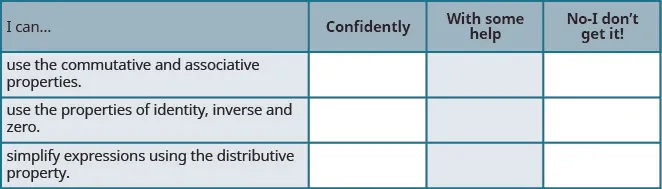After reviewing this checklist, what will you do to become confident for all objectives?

Order a print copy

As an Amazon Associate we earn from qualifying purchases.